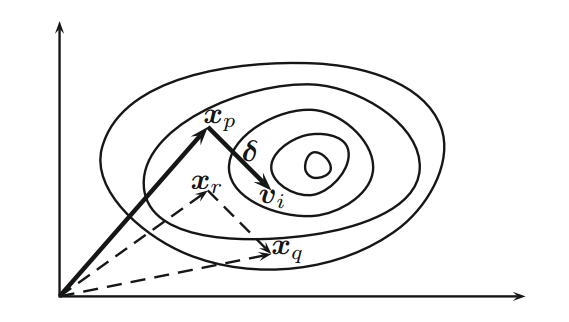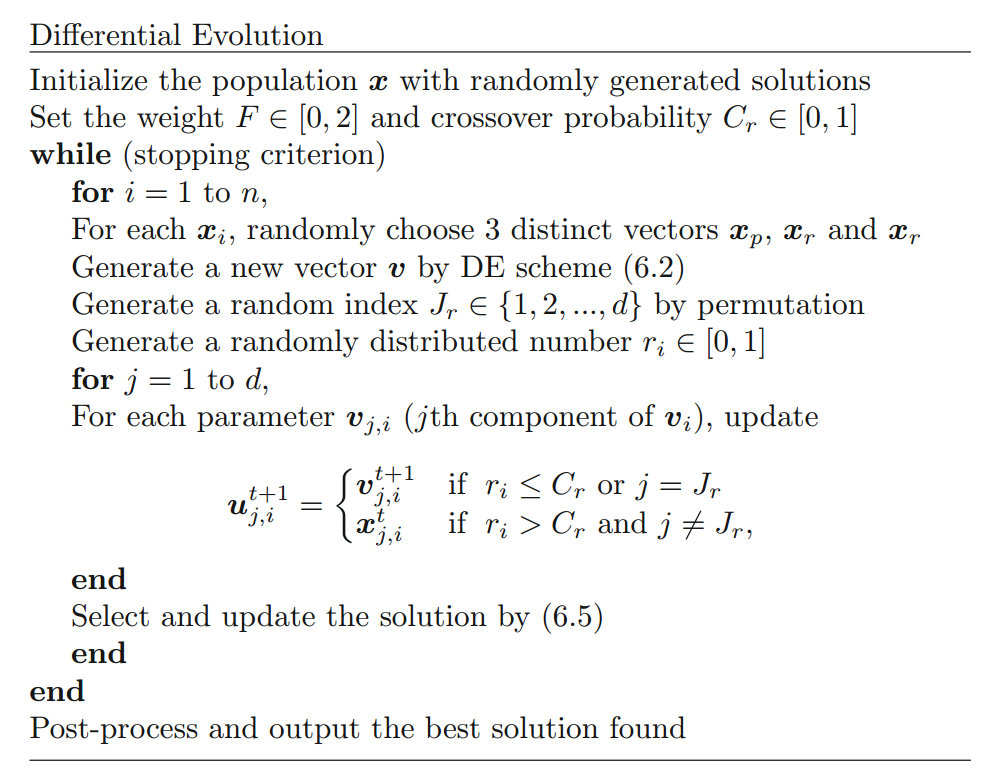## 差分进化

$$x_i^t = (x_{1,i}^t, x_{2,i}^t, … , x_{d, i}^t), \tag 1$$

$$v_i^{t+1} = x_p^t + F(x_q^t - x_r^t), \tag 2$$Figure 1 Schematic representation of mutation vectors in differential evolution with movement $\delta=F(x_q - x_r)$.

$$u_{j, i}^{t+1} = \begin{cases} v_{j, i} & \text{if r_i \le C_r}, \ x_{j, i}^t & \text{otherwise}, \end{cases} j = 1, 2, … , d. \tag 3$$

$$u_{j, i}^{t+1} = \begin{cases} v_{j, i}^t & \text{for j=k,…,k-L+1 \in [1, d]}, \ x_{j, i}^t & \text{otherwise}. \end{cases} \tag 4$$

$$x_i^{t=1} = \begin{cases} u_i^{t+1} & \text{if f(u_i^{t+1}) \le f(x_i^t)}, \ x_i^t & \text{otherwise}. \end{cases} \tag 5$$Figure 2 Pseudo code of differential evolution.

## 算法变体

$$v_i^{t+1} = x_p^t + F(x_q^t - x_r^t), \tag 6$$

$$v_i^{t+1} = x_{best}^t + F(x_q^t - x_r^t). \tag 7$$

$$v_i^{t+1} = x_{best}^t + F(x_{k1}^t + x_{k2}^t - x_{k3}^t - x_{k4}^t). \tag 8$$

$$v_i^{t+1} = x_{k1}^t + F_1(x_{k2}^t - x_{k3}^t) + F_2(x_{k4}^t - x_{k5}^t), \tag 9$$

$$v_i^{t+1} = x_{k1}^t + \sum_{s=1}^m F_s \cdot (x_{k2(s)}^t - x_{k3(s)}^t), \tag {10}$$

$$v_i^{t+1} = \lambda x_{best}^t + (1 - \lambda)x_{k1}^t + F(x_{k2}^t - x_{k3}^t), \tag {11}$$

$$v_i^{t+1} = \lambda x_{best}^t + (1 - \lambda)x_{k1}^t + F \sum_{s=1}^m (x_{k2(s)}^t - x_{k3(s)}^t). \tag {12}$$

## 收敛性分析

$$2mF^2 + (1 - \lambda)^2 \gt 1, \lambda \in (0, 1). \tag {13}$$

$$E(var(P)) = c , var(X), \tag {14}$$

$$E(var(P)) = (1 + 2p_mF^2 - \frac{p_m(2 - p_m)}{n})var(X), \tag {15}$$

$$p_m = \begin{cases} C_r(1 - \frac 1d) + \frac 1d & \text{(binomial crossover)}, \ \frac{1 - C_r^d}{d(1 - C_r)} & \text{(exponential crossover)}. \end{cases} \tag {16}$$

$$var(P) = (1 + 2F^2p_m - \frac{p_m(2-p_m)}{n})^tvar(X(0)). \tag {17}$$

$$1 + 2F^2p_m - \frac{p_m(2 - p_m)}{n} = 1, \tag {18}$$

$$F_c = \sqrt{\frac{(2 - p_m)}{n}}. \tag {19}$$

$$p_m = C_r (1 - \frac 1d) + \frac 1d = 0.5 \times (1 - \frac{1}{10}) + \frac{1}{10} = 0.55. \tag {20}$$

$$C_r = \frac {(p_m - \frac 1d)}{(1 - \frac 1d)}. \tag {21}$$

$$C_r = \frac{(0.2 - 1/10)}{(1 - 1/10)} \approx 0.44. \tag {22}$$

## 实现

TianYuanSX 在代码中增加了迭代次数上限以及新的停止条件，改进的代码如下所示：

 Beyer HG. On the dynamics of EAs without selection. In: Banzaf W, Reeves C, editors. Foundations of genetic algorithms. San Francisco, CA, USA: Morgan Kaufmann Publishers; 1999. p. 5–26.

 Brest J, Greiner S, Boskovic B, Mernik M, Zumer V. Self-adapting control parameters in differential evolution: a comparative study on numerical benchmark functions. IEEE Trans Evol Comput 2006;10(6):646–57.

 Chakraborty UK. Advances in differential evolution. Studies in computational intelligence, vol. 143. Heidelberg, Germany: Springer; 2008.

 Das S, Suganthan PN. Differential evolution: a survey of the state-of-the-art. IEEE Trans Evol Comput 2011;15(1):4–31.

 Jeyakumar G, Velayutham CS. Convergence analysis of differential evolution variants on unconstrained global optimization functions. Int J Art Intell Appl 2011;2(2):116–26.

 Qin AK, Huang VL, Suganthan PN. Differential evolution algorithm with strategy adaptation for global numerical optimization. IEEE Trans Evol Comput 2009;13(2):398–417.

 Storn R. On the usage of differential evolution for function optimization. In: Biennial conference of the North American fuzzy information processing society (NAFIPS); 1996. p. 519–23.

 Storn R, Price K. Differential evolution: a simple and efficient heuristic for global optimization over continuous spaces. J Global Optimization 1997;11:341–59.

 Price K, Storn R, Lampinen J. Differential evolution: a practical approach to global optimization. Germany: Springer; 2005.

 Xue F, Sanderson AC, Graves RJ. Multiobjective differential evolution: algorithm, convergence analysis and applications. In: Proceedings of congress on evolutionary computation (CEC 2005), Edinburgh, vol. 1. Edinburgh, Scotland: IEEE Publication; 2005. p. 743–50.

 Yang XS, Deb S. Two-stage eagle strategy with differential evolution. Int J Bio-Inspired Comput 2012;4(1):1–5.

 Zaharie D. A comparative analysis of crossover variants in differential evolution. In: Ganzha M, Paprzycki M, Pilichowski TP, editors. Proceedings of the international multiconferenceon computer science and information technology, October 15–17, 2007. Poland: Wisla; 2007. p. 171–81. (Polskie Towarzystwo Informatyczne, PIPS, Katowice).

 Zaharie D. Influence of crossover on the behavior of the differential evolution algorithm. Appl Soft Comput 2009;9(3):1126–38.

 Zaharie D. Differential evolution: from theoretical results to practical insights. In: Matousek R, editor. Proceedings of the 18th international conference on soft computing, June 27–29, Brno. Czech Republic: Brno University of Technology Press; 2012. p. 126–31.